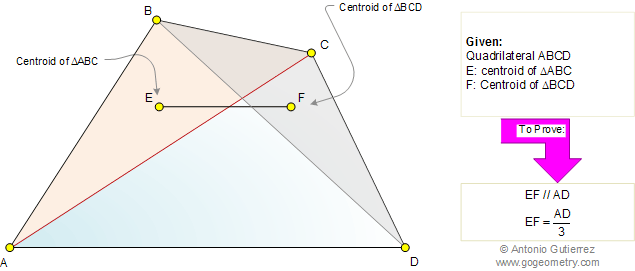Home Geometry Problems All problems Visual Index Centroid 571-580 Post a solution        By Antonio Gutierrez
 Problem 576: Centroid, Triangle, Quadrilateral, Parallel, Measurement. Level: High School, College, Math Education. The figure shows a quadrilateral ABCD. E is the centroid of triangle ABC and F is the centroid of triangle BCD. Prove that (1) EF is parallel to AD, (2) the measure of EF is one-third of the measure of AD.Recent Additions